# 第 36 章 基於秩次的非參數檢驗

## 36.1 符號檢驗 the Sign test

n=24 Diabetics
10.3 8.8 5.3
9.5 6.7 6.7
12.2 12.5 5.2
15.1 4.2 13.3
10.8 15.3 7.5
19.0 7.2 4.9
16.1 9.3 19.5
8.1 8.6 11.1

$H_0: \theta=\theta_0 \\ H_1: \theta\neq\theta_0$

$X\sim Bin(n, 0.5)$

$2\times P(X\leqslant x|\pi=0.5)$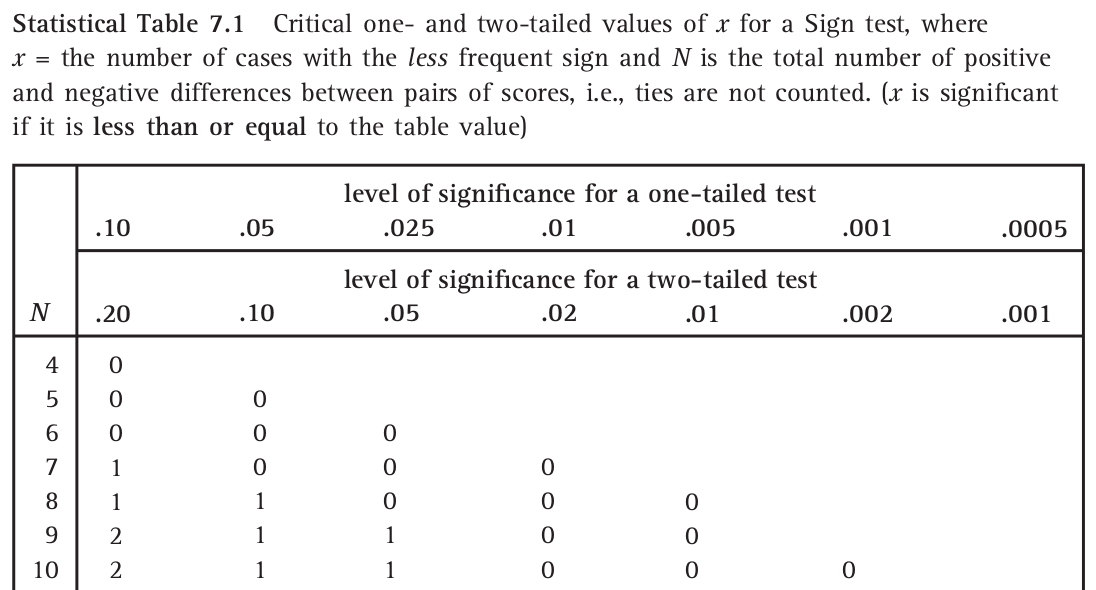$\cdots\cdots\cdots$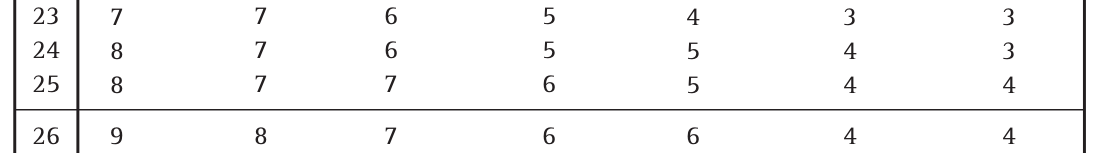2*pbinom(11,24, 0.5)
##  0.8388

options(scipen = 1, digits = 8) # just to show the p values are exactly the same
binom.test(11,24,0.5)
##
##  Exact binomial test
##
## data:  11 and 24
## number of successes = 11, number of trials = 24, p-value = 0.83882
## alternative hypothesis: true probability of success is not equal to 0.5
## 95 percent confidence interval:
##  0.25553020 0.67179192
## sample estimates:
## probability of success
##             0.45833333

options(scipen = 1, digits = 8)
# input the data
dt <- c(10.3,9.5,12.2,15.1,10.8,19.0,16.1, 8.1, 8.8, 6.7,12.5, 4.2,15.3, 7.2, 9.3, 8.6, 5.3,6.7 ,5.2,13.3, 7.5, 4.9,19.5,11.1)
BSDA::SIGN.test(dt, md=10, alternative="two.sided", conf.level=0.95)
##
##  One-sample Sign-Test
##
## data:  dt
## s = 11, p-value = 0.83882
## alternative hypothesis: true median is not equal to 10
## 95 percent confidence interval:
##   7.3988241 12.3011759
## sample estimates:
## median of x
##         9.4
##
## Achieved and Interpolated Confidence Intervals:
##
##                   Conf.Level L.E.pt  U.E.pt
## Lower Achieved CI     0.9361 7.5000 12.2000
## Interpolated CI       0.9500 7.3988 12.3012
## Upper Achieved CI     0.9773 7.2000 12.5000

“據說”如果你用 Stata 的話還會給你一個絢麗的表格：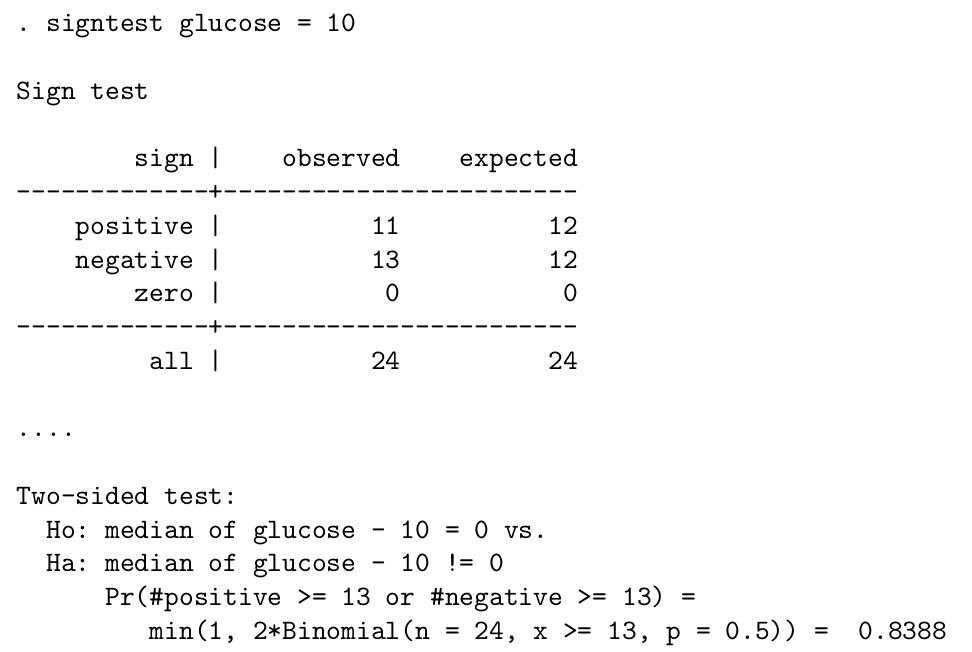$z=\frac{\frac{x}{n}-\pi}{\sqrt{\pi(1-\pi)/n}}=\frac{\frac{x}{n}-0.5}{\sqrt{0.5(1-0.5)/n}}=\frac{2x}{\sqrt{n}}-\sqrt{n}\\ \text{With the continuity correction, this becomes: }\\ z=\lvert\frac{2x}{\sqrt{n}}-\sqrt{n}\rvert-\frac{1}{\sqrt{n}}$

$z=\lvert\frac{2\times11}{\sqrt{24}}-\sqrt{24}\rvert-\frac{1}{\sqrt{24}}=0.204$

(1-pnorm(0.204))*2
##  0.8383535

## 36.2 Wilcoxon 符號秩和檢驗，the Wilcoxon signed-rank test

Wilcoxon 符號秩和檢驗也可以用來檢驗一組數據的中位數是否和某個已知數字相等。進行本方法時除了像符號檢驗那樣要假設數據是連續的以外，還要假設數據的分佈是左右對稱的。因此，由於假定了左右對稱的前提，中位數也就等於均數，所以它也可以被用於檢驗均數是否等於某個已知的值。

Wilcoxon 符號秩和檢驗的前提可以被認爲是介於符號檢驗和單一樣本 $$t$$ 檢驗 (還假設數據來自於正態分佈) 之間的一種檢驗。

1. 將全部觀察數據一一和 $$\theta_0$$ 相減，那麼每一個 $$d_i=x_i-\theta_0, i=1,2,\cdots,n$$ 的正負符號概率是相等的；
2. 任何一個和 $$\theta_0$$ 相減之後的差 $$\lvert d_i \lvert$$ ，取正負符號的概率是相等的。

-2, -5, 12, 4, 16, 17, 8, 3, 20, 25, 1, 9

data <- c(-2, -5, 12, 4, 16, 17, 8, 3, 20, 25, 1, 9)
newdata <- data-15
newdata
##   -17 -20  -3 -11   1   2  -7 -12   5  10 -14  -6

abs_newdata <- abs(newdata)
abs_newdata
##   17 20  3 11  1  2  7 12  5 10 14  6
Dt <- data.frame(data, newdata, abs_newdata)
Dt <- Dt[order(newdata), ] # sort the data by newdata
Dt
##    data newdata abs_newdata
## 2    -5     -20          20
## 1    -2     -17          17
## 11    1     -14          14
## 8     3     -12          12
## 4     4     -11          11
## 7     8      -7           7
## 12    9      -6           6
## 3    12      -3           3
## 5    16       1           1
## 6    17       2           2
## 9    20       5           5
## 10   25      10          10

Dt$ranks <- rank(Dt$abs_newdata)
Dt
##    data newdata abs_newdata ranks
## 2    -5     -20          20    12
## 1    -2     -17          17    11
## 11    1     -14          14    10
## 8     3     -12          12     9
## 4     4     -11          11     8
## 7     8      -7           7     6
## 12    9      -6           6     5
## 3    12      -3           3     3
## 5    16       1           1     1
## 6    17       2           2     2
## 9    20       5           5     4
## 10   25      10          10     7

Dt$signed_ranks <- ifelse(Dt$newdata < 0, Dt$ranks*(-1), Dt$ranks)
Dt <- Dt[order(Dt$ranks),] Dt ## data newdata abs_newdata ranks signed_ranks ## 5 16 1 1 1 1 ## 6 17 2 2 2 2 ## 3 12 -3 3 3 -3 ## 9 20 5 5 4 4 ## 12 9 -6 6 5 -5 ## 7 8 -7 7 6 -6 ## 10 25 10 10 7 7 ## 4 4 -11 11 8 -8 ## 8 3 -12 12 9 -9 ## 11 1 -14 14 10 -10 ## 1 -2 -17 17 11 -11 ## 2 -5 -20 20 12 -12 對正的負的 signed_ranks 分別求和，絕對值較小的那個就是 Wilcoxon 檢驗的統計量。本例中：$$S^+=$$ 14，$$S^-=$$ -64，所以本例中的檢驗統計量等於 $$14$$。如果要繼續查表的話，可以找到 $$0.05<p<0.1$$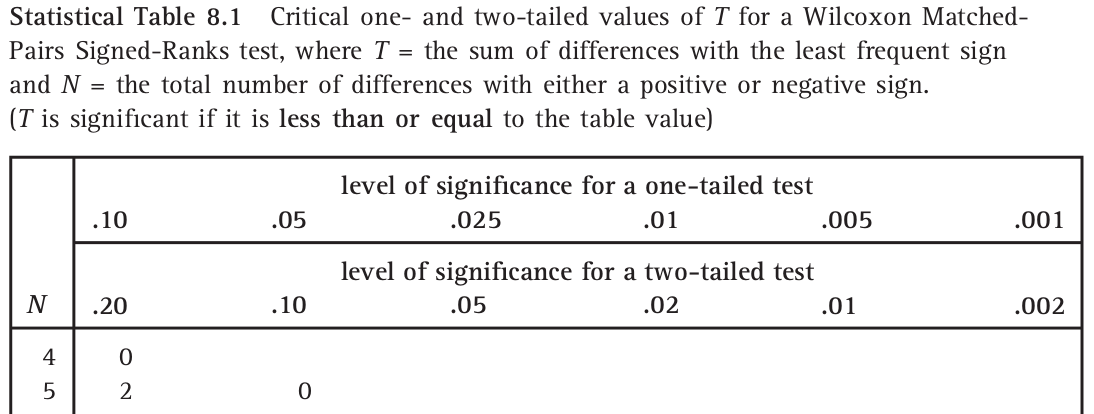$\cdots\cdots\cdots$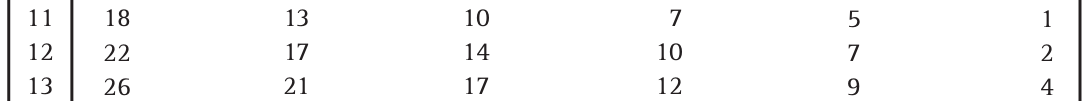圖 36.3: Critical Values for a Wilcoxon Signed-Ranks test 精確的 Wilcoxon 符號秩和檢驗可以通過下列代碼在 R 裏完成： wilcox.test(Dt$data, mu=15, paired = FALSE)
##
##  Wilcoxon signed rank test
##
## data:  Dt$data ## V = 14, p-value = 0.052246 ## alternative hypothesis: true location is not equal to 15 如果數據中有觀察值和我們希望比較的數值完全相等的話，和符號檢驗類似的，這些觀察值需要被剔除之後再進行上面的個步驟檢驗。記得還要將樣本量減去相應個數再去查表尋找 $$p$$ 值。 另外，下面的代碼可以計算正態分佈近似的 Wilcoxon 秩和檢驗，結果十分接近： wilcox.test(Dt$data, mu=15, paired = FALSE, exact = FALSE, correct = FALSE)
##
##  Wilcoxon signed rank test
##
## data:  Dt$data ## V = 14, p-value = 0.04986 ## alternative hypothesis: true location is not equal to 15 值得注意的是，精確計算時，我們需要剔除那些和比較數值完全一致的觀察值。然而在正態分佈近似法的 Wilcoxon 檢驗中，這些數值並不會被剔除，而是保留下來，並且用於對方差進行調整。Wilcoxon 秩和檢驗可以在我們能夠假設數據左右對稱分佈，且明顯不服從正態分佈時使用 (即概率密度分布圖左右兩端的尾部較厚的時候)。如果數據左右完全對稱，本檢驗方法不太推薦採用。 ## 36.3 Wilcoxon-Mann-Whitney (WMW) 檢驗 本方法用於比較兩組獨立樣本的分佈是否只是左右位移 (或者叫平移)。此檢驗需要的假設前提爲：兩組獨立樣本來自連續型分佈，且僅僅只存在整體的左右位移 (或者叫平移) (location shift)。 例如說，兩組數據各自的累積概率方程分別是 $$F(\cdot), G(\cdot)$$ 時，由上面的假設可知： $G(y)=F(y-\Delta), \text{ where } -\infty<\Delta<\infty$ 上面式子中的 $$\Delta$$ 就是所謂的 “左右位移 (或者叫平移)”。因此本檢驗的零假設和替代假設爲： $H_0: \Delta=0\\ H_1: \Delta\neq0$ 在零假設的條件下，我們可以認爲兩個樣本來自相同的人羣分佈。假如，$$F, G$$ 都是正態分佈，且同方差。那麼 $$\Delta$$ 就等於兩個分佈的均值差。在這種情況下就可以使用兩樣本 $$t$$ 檢驗。所以說，WMW 檢驗其實就是把假設前提放寬了的 (免去了正態分佈假設) 兩樣本 $$t$$ 檢驗。 如果兩個獨立樣本分別有樣本量 $$n, m, \text{ and } n<m$$$$X_1,\cdots,X_n$$$$Y_1, \cdots, Y_m$$。在零假設的條件下，將這兩個樣本合併之後的大樣本 ($$m+n$$ 個樣本量) ：$$X_1,\cdots,X_n,Y_1, \cdots, Y_m$$ 可以視爲來自同一分佈。那麼在合併後的樣本中，我們給每一個元素賦予它們的合併後數據中的排序 ($$\text{Rank}_i: i= 1,2,\cdots,n, n+1, \cdots, n+m$$)。那麼我們感興趣的 Wilcoxon 秩和統計量 (Wilcoxon rank sum statistic) $$W_1$$ 是樣本量較小的那些數字在合併後數據中的排序之和。 $W_1=\sum_{i=1}^n R_i$ 對於大小相同的數據，排序取他們的排序的平均值。 之後再計算 ： $U_1=W_1+\frac{n(n+1)}{2}$ 最後拿來判斷的檢驗統計量是 $$U_1$$$$n\times m -U_1$$ 兩者中較小的數字。(注：如果我們一開始計算樣本量較多的部分的秩和 $$W_2=\sum_{i=1}^m R_i$$ 時，將計算的 $$U_2=W_2-\frac{m(m+1)}{2}$$，跟 $$n\times m - U_2$$ 中較小的數字作爲檢驗統計量的話，我們會在數學上獲得完全一樣的檢驗統計量。) 其實兩個統計量 $$W, U$$ 均可以用來作相同的統計推斷，當然各自的 $$p$$ 值表格不同。但是 $$U$$ 有另外一種統計學含義：$$U$$$$X_i>Y_j$$， 也就是所有的 $$(X_i, Y_j)$$ 配對中 $$X_i$$ 較大的對的個數。 這裏使用下面的例子來解釋如何操作 WMW 檢驗： 採集16名甲亢兒童的血清甲狀腺素濃度值列表如下， 表 36.2: Serum thyroxine levels n=16 hypothyroid children thyr group 34 Slight or no symptoms 45 Slight or no symptoms 49 Slight or no symptoms 55 Slight or no symptoms 58 Slight or no symptoms 59 Slight or no symptoms 60 Slight or no symptoms 62 Slight or no symptoms 86 Slight or no symptoms 5 Marked symptoms 8 Marked symptoms 18 Marked symptoms 24 Marked symptoms 60 Marked symptoms 84 Marked symptoms 96 Marked symptoms 這裏我們需要比較輕微症狀組和嚴重症狀組的血清甲狀腺濃度的分佈是否只是左右位移，$$H_0: \Delta=0$$ 首先，我們要給兩組合併後的濃度排序： dt$rank <- rank(dt$thyr) dt <- dt[order(dt$rank),]
dt
##    thyr                 group rank
## 10    5       Marked symptoms  1.0
## 11    8       Marked symptoms  2.0
## 12   18       Marked symptoms  3.0
## 13   24       Marked symptoms  4.0
## 1    34 Slight or no symptoms  5.0
## 2    45 Slight or no symptoms  6.0
## 3    49 Slight or no symptoms  7.0
## 4    55 Slight or no symptoms  8.0
## 5    58 Slight or no symptoms  9.0
## 6    59 Slight or no symptoms 10.0
## 7    60 Slight or no symptoms 11.5
## 14   60       Marked symptoms 11.5
## 8    62 Slight or no symptoms 13.0
## 15   84       Marked symptoms 14.0
## 9    86 Slight or no symptoms 15.0
## 16   96       Marked symptoms 16.0

Wilcoxon 統計量 $$W_1$$ 是人數少的組的排序之數值和。本樣本中 7 人有嚴重症狀，9人有輕微或無症狀。所以 $$W_1$$ 就是嚴重症狀組的秩和：

$W_1=1+2+3+4+11.5+14+16=51.5$

$U_1=W_1-\frac{n(n+1)}{2}=51.5-\frac{7\times(7+1)}{2}=23.5$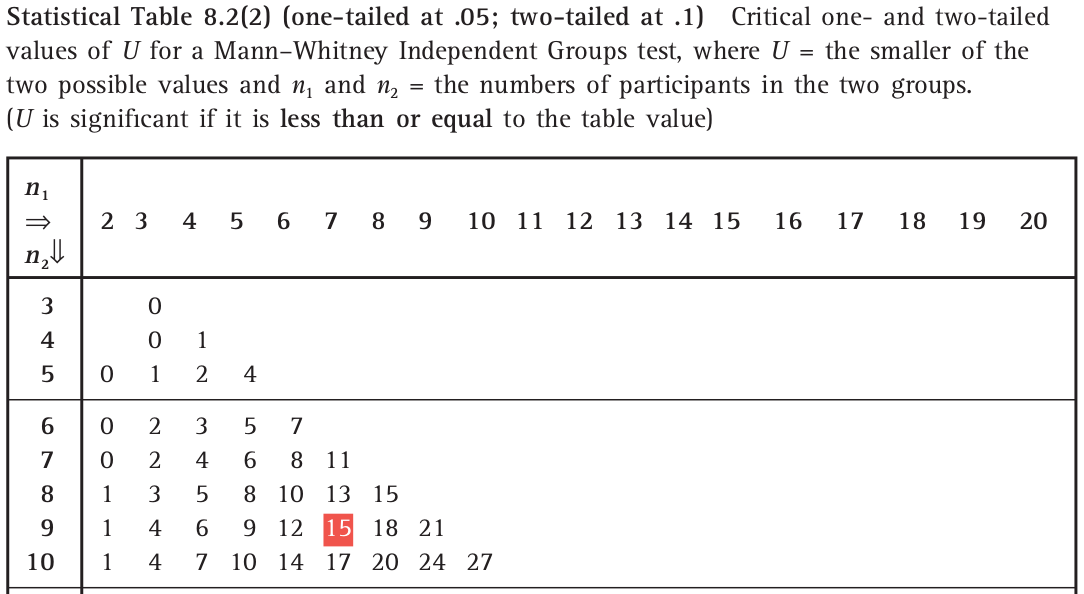R 裏面用下面的代碼進行 WHW 檢驗：

wilcox.test(dt$thyr~dt$group, correct=FALSE) # without continuity correction
##
##  Wilcoxon rank sum test
##
## data:  dt$thyr by dt$group
## W = 23.5, p-value = 0.39675
## alternative hypothesis: true location shift is not equal to 0
wilcox.test(dt$thyr~dt$group) # with continuity correction i.e. normal appriximation
##
##  Wilcoxon rank sum test with continuity correction
##
## data:  dt$thyr by dt$group
## W = 23.5, p-value = 0.42692
## alternative hypothesis: true location shift is not equal to 0

## 36.4 秩相關，Spearman’s Rank Correlation Coefficient

Spearman 的秩相關 (通常用 $$\rho$$)，是一種基於數據排序的相關係數算法。和傳統的 Pearson 相關係數類比，是當數據無法被認定是線性相關時的另一種相關關係檢驗方法。所以秩相關不假定兩組數據之間是線性相關 (linear association)。秩相關只關心一個數據遞增時，另一個數據是否單調遞增。所以可以用於傾向性檢驗。

$\hat\tau=\frac{\hat\rho}{\sqrt{(1-\hat\rho^2)/(n-2)}}$

n=28 haemophiliacs
th4 th8
0.20 0.17
0.27 0.52
0.28 0.25
0.37 0.34
0.38 0.14
0.48 0.10
0.49 0.58
0.56 0.23
0.60 0.24
0.64 0.67
0.64 0.90
0.66 0.26
0.70 0.61
0.77 0.18
0.88 0.74
0.88 0.54
0.88 0.76
0.90 0.62
1.02 0.48
1.10 0.58
1.10 0.34
1.18 0.84
1.20 0.63
1.30 0.46
1.40 0.84
1.60 1.20
1.64 0.59
2.40 1.30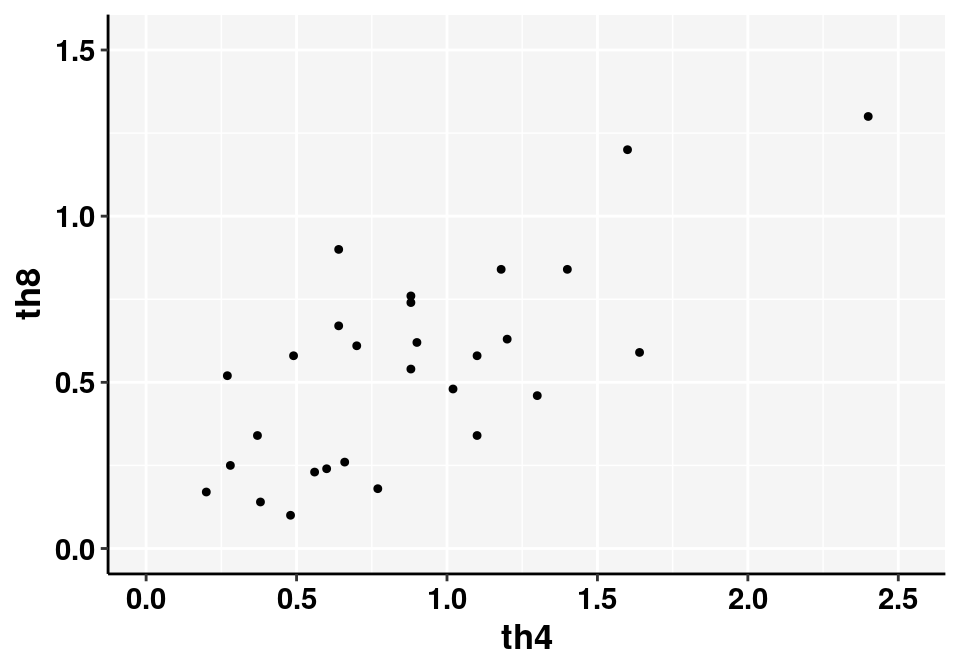dt$rank4 <- rank(dt$th4)
dt$rank8 <- rank(dt$th8)
kable(dt, "html", align = "c",caption = "Lymphocyte counts") %>%
kable_styling(bootstrap_options = c("striped", "bordered")) %>%
#  collapse_rows(columns = c(1)) %>%
scroll_box(width = "400px", height = "500px", extra_css="margin-left: auto; margin-right: auto;")

n=28 haemophiliacs with ranks
th4 th8 rank4 rank8
0.20 0.17 1.0 3.0
0.27 0.52 2.0 13.0
0.28 0.25 3.0 7.0
0.37 0.34 4.0 9.5
0.38 0.14 5.0 2.0
0.48 0.10 6.0 1.0
0.49 0.58 7.0 15.5
0.56 0.23 8.0 5.0
0.60 0.24 9.0 6.0
0.64 0.67 10.5 21.0
0.64 0.90 10.5 26.0
0.66 0.26 12.0 8.0
0.70 0.61 13.0 18.0
0.77 0.18 14.0 4.0
0.88 0.74 16.0 22.0
0.88 0.54 16.0 14.0
0.88 0.76 16.0 23.0
0.90 0.62 18.0 19.0
1.02 0.48 19.0 12.0
1.10 0.58 20.5 15.5
1.10 0.34 20.5 9.5
1.18 0.84 22.0 24.5
1.20 0.63 23.0 20.0
1.30 0.46 24.0 11.0
1.40 0.84 25.0 24.5
1.60 1.20 26.0 27.0
1.64 0.59 27.0 17.0
2.40 1.30 28.0 28.0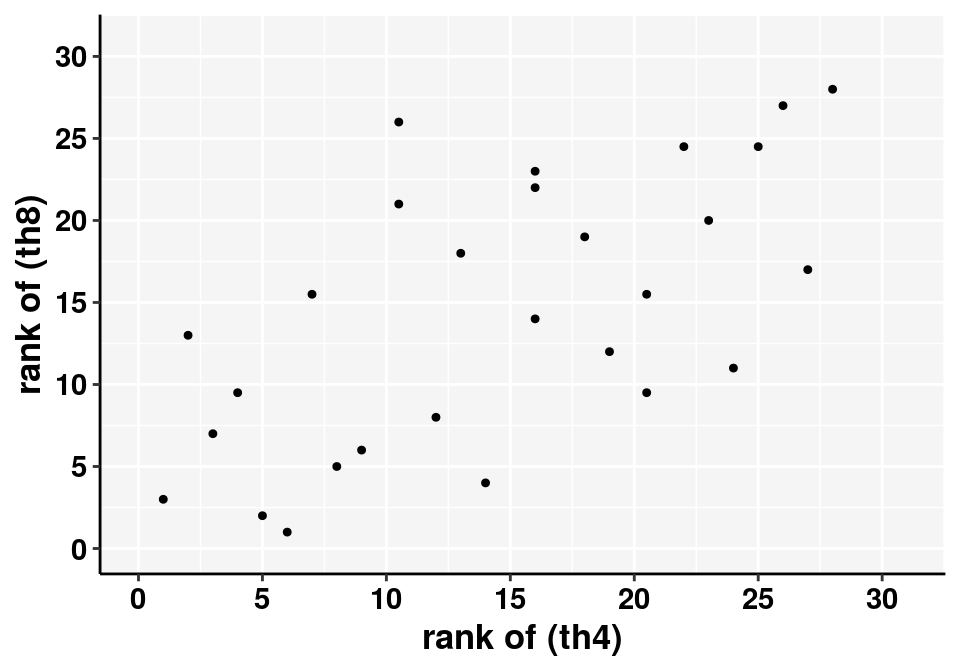cor.test(dt$rank4, dt$rank8)
##
##  Pearson's product-moment correlation
##
## data:  dt$rank4 and dt$rank8
## t = 4.11513, df = 26, p-value = 0.00034606
## alternative hypothesis: true correlation is not equal to 0
## 95 percent confidence interval:
##  0.33297073 0.81107104
## sample estimates:
##        cor
## 0.62803129
cor.test(dt$th4, dt$th8)
##
##  Pearson's product-moment correlation
##
## data:  dt$th4 and dt$th8
## t = 5.28031, df = 26, p-value = 0.000016061
## alternative hypothesis: true correlation is not equal to 0
## 95 percent confidence interval:
##  0.47328797 0.86128090
## sample estimates:
##        cor
## 0.71934766

## 36.5 基於秩次的非參數檢驗的優缺點

• 可以讓我們拋棄很多 (正態分佈等的) 前提假設，許多真實數據本身並不能滿足這些條件，這些情況下，基於秩次的非參數檢驗能提供更高的統計效能 (power)。

• 如果數據本身能夠滿足如正態分佈之類的假設，那麼相比較與一般的參數檢驗，基於秩次的非參數檢驗法效能就偏低。
• 基於秩次的非參數檢驗較難推廣到更加複雜的情況。
• 這些檢驗法僅僅只能幫助我們進行假設檢驗。但是多數情況下，我們更加希望能使用觀察數據對總體進行點估計 (point estimates) 並且給出信賴區間 (CIs)。通常情況下，基於秩次的非參數檢驗法就很難給出這樣的估計。